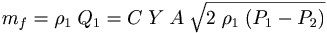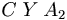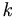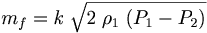Mass Flow by square root extraction

Measuring the flow of fluids with an orifice plate

Sometimes we want to measure the flow of a material that isn't happy going through an impeller (or we might want to avoid having to maintain the shaft seal). One way to accomplish this is with a differential pressure transducer that looks at the difference of pressure across an orifice plate.

We can get volume or mass flow using the following equationwhere

Q1 = upstream volumetric flow, m³/s
mf = mass flow rate at any point, kg/s
C = orifice flow coefficient
Y = expansion factor
A = cross-sectional area of orifice, m²
P1 = upstream pressure, Pa   kg/(m ·s)
P2 = downstream pressure, Pa   kg/(m ·s)
ρ1 = upstream fluid density, kg/m³

In practice ,become a lumped constant along with any unit scaling- Let's call this constant ,thenThe flow is a constant times the square root of a constant times the pressure difference.

Panel meters often can figure out ,and ρ1 by looking at two known flow rates.

 Top Page wiki Index

Disclaimer

This information may have errors; It is not permissible to be read by anyone who has ever met a lawyer.
Use is confined to Engineers with more than 370 course hours of electronic engineering for theoretical studies.
ph +1(785) 841-3089